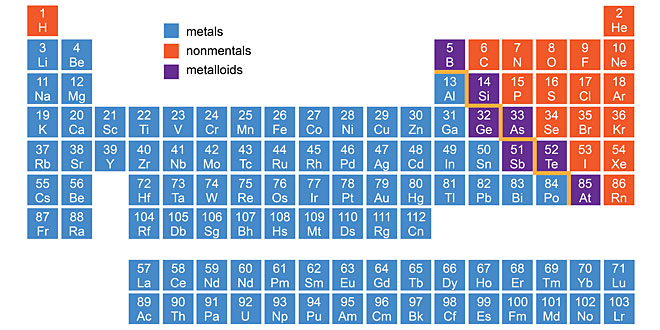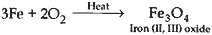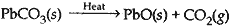Saturday , January 25 2020
Home / 10th Class / Metals and Non-metals: 10th Science# Metals and Non-metals: 10th Science

#### Question: Explain, why calcium metal after reacting with water starts floating on its surface. Write the chemical equation.

Answer: Calcium starts floating because the bubbles of hydrogen gas formed stick to the surface of the metal.

Ca(s)   + 2H2O(l)  →  Ca(OH)2(aq)     +     H2(g)
(Calcium)     (water)             (Calcium hydroxide)        (Bubbles of hydrogen gas)

#### Question: When a metal X is treated with cold water, it gives a basic salt Y with molecular formula XOH (Molecular mass = 40) and liberates a gas Z which easily catches fire. Identify X, Y, Z.

1. X is sodium or potassium metal.
2. Y is sodium hydroxide or potassium hydroxide.
3. Z is hydrogen gas.

#### Question: Name the following: (1). A metal which is preserved in kerosene. (2). A lustrous colored non-metal. (3). A metal which can melt while kept on palm. (4). A metal which is a poor conductor of heat.

1. Sodium
2. Iodine
3. Gallium or caesium

#### Question: Why do ionic compounds have high melting points? State reason.

Answer: In ionic compounds, cations are held together by strong electrostatic forces of attraction. Hence, large amount of energy is required to overcome these forces.

#### Question: Write chemical equations for the reactions taking place when: (1) iron reacts with steam. (2) magnesium (mg) reacts with dil. HCl. (3) copper is heated in air.

1. 3Fe(s) + 4H2O(g) → Fe3O4(s) + 4H2(g)
2. Mg      +      2HCl     →    MgCl2         +      H2
Magnesium                 Magnesium chloride
3. 2Cu(s) + O2(g) → 2CuO
Copper II oxide

#### Question: You are given samples of three metals – sodium, magnesium and copper. Suggest any two activities to arrange them on order of their decreasing reactivity.

Activity 1:
Sodium reacts with cold water to form sodium hydroxide and hydrogen gas.
2Na(s) + 2H2O(l) → 2NaOH(aq) + H2(g)
Magnesium does not react with cold water but with hot water to form magnesium hydroxide and hydrogen gas.
Mg + 2H2O → Mg(OH)2 + H2
(Hot)
Hence, sodium is more reactive than magnesium.

Activity 2:
Mg + CuSO4  → MgSO4 + Cu
Cu + MgSO4  → No reaction
So magnesium is more reactive than copper. Concluding from activity 1 and activity 2, Na > Mg > Cu.

#### Question: Name two metals in each of the following cases: (1). Metals that are stored in kerosene. (2). Metals that are alloyed with iron to make stainless steel. (3). Metals which are highly malleable and ductile.

1. Sodium and potassium
2. Nickel and chromium
3. Gold and silver

#### Question: Write chemical equations for the reaction taking place when: (i). Iron is strongly heated in air. (ii). lead carbonate is calcined. (iii). chromium oxide (Cr2O3) is heated with aluminium powder.

(i). Iron does not burn in air even on strong heating. At high temperature, it combines with oxygen of the air to form Fe3O4.(ii). Calcination of lead carbonate. Heating the ore in absence of air.(iii) Cr2O3(s) + Al(s) → Al2O3(s) + 2Cr(l
Chromium is obtained in molten form.

#### Question: A, B and C are three elements which undergo chemical reactions according to the following equations: A2O3  + 2B → B2O3 + 2A 3CSO4  +  2B  →  B2(SO4)3 + 3C 3CO + 2A → A2O3 + 3C (1) Which element is the most reactive? (2) Which element is the least reactive? (3) What is the type of reactions listed above?

1. Most reactive element is ‘B’ as it has replaced both ‘A’ and ‘C’ from their compounds.
2. Element ‘C’ is least reactive as it has been replaced by both ‘A’ and ‘B’.
3. Displacement reaction.